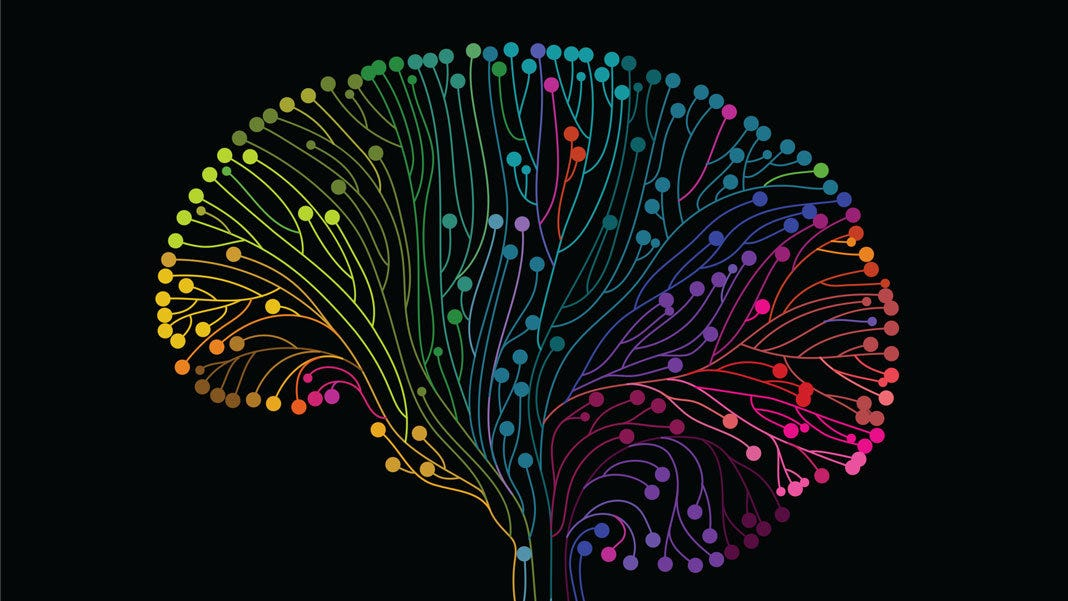# Different Optimization Algorithm for Deep Neural Networks: Complete GuideIn this article, we will only focus on the Better Optimizing algorithm for Deep Neural Network (DNN). There are several well-known Optimizing algorithms out there. We will call this optimizing algorithm as Learning algorithm for this article. let’s have a look at them.

## Momentum-Based Learning Algorithm

3. Nesterov Accelerated Gradient Descent (NAG)

## Batch-Learning Based Learning Algorithm

1. Stochastic Update
2. Mini-Batch Update

## Adaptive Learning rate Based Learning Algorithm

2. RMS Prop
3. Adam (a mixture of RMS Prop and Momentum based GD)

# Momentum-Based Learning Algorithm

Gradient descent is one of the most popular and most used learning algorithms to perform optimization and the oldest technique to optimize neural networks. It is an algorithm which is mostly inspired by the famous technique “local minimum” or “hill-climbing”. It’s first introduced in 1944.

Now let’s have a look at the basic intuition of the Gradient Descent algorithm.

Say you’re walking down the valley, your goal is to reach the local minimum place. But from your current position, you can not jump off to that position or you are unable to view your goal. In every position, you have to make a decision based on your current position.

• You might go upward or downward
• Your step size depends on two things, the slope of your current position that is descent and predefined step size (learning rate).

Now you can overshoot your goal if you have a larger step size. But if you have a very small step size, it’ll take a lot of time to reach the desired goal state. So you have to balance between them to get a better result.

Now, this is the basic Intuition of Gradient Decent approach we will see more in different GD based learning algorithms.

Now let’s see the main motivation of the Vanilla Gradient descent algorithm, it’s a basic GD algorithm without any modification.

Here in the fig. the red color denotes high loss, blue zone denotes the minimum loss. Say, we are starting our journey from A and our goal is to reach the global minimum in a minimal number of steps.

let’s have a look at the main update rule for Gradient Descent.

we will discuss the loss function in another article, for now, we will focus only on the main function.

Here the main idea is by computing the derivative/slope of the function, we can find the minimum of a function. And the learning rate will help us to set the step size. More, we get closer to the global minimum, The loss function L(w) will get minimum and that’s why our update will be minimum.

let’s have a look at the code part :

Here X is the data point and Y is the corresponding label.

Pros:
1. Easy to implement

Cons:
1. The algorithm takes an entire dataset in one go and computes the derivative to update the weight which requires a lot of memory and time.
2. The algorithm can be stuck at local minima or saddle point

Now after the GD algorithm, we need to think about two questions.

1. How do you use the gradients?
we are using just the current gradient to update the previous weight values.
2. Can we come up with a better update rule than this?
can we use the previous gradient with the current gradient together to form a better update rule? I think that will be a good idea. And it’s called Momentum based Gradient Descent. Where we calculate momentum based on prev gradients.

let’s have a look at them:

Now clearly, from the above equation if add γ=0 we will get vanilla GD equation i.e if we don’t want to add history.

Now let’s have a look at the code:

Now we can clearly see it’s coming out of that local minimum and even reaching the global minimum in 700 epochs.

Pros:
1. Reduce the oscillations and high variance of parameters.
2. Converges faster than Gradient Descent

Cons:
1. Even now it’s taking longer to converge because it moving so fast, so it might take some wrong turn.

if we plot the graph in 2D then we can see, in the last part it is overshooting the global minimum and there is some oscillation.
2. One more Hyper-parameter added, which needs to be selected manually and accurately.

## Nesterov Accelerated Gradient Descent (NAG)

So momentum-based GD was quite good, but there are still some disadvantages. Is moving fast is always good? how we can rectify it.

Nesterov Accelerated Gradient Descent someway fixed these difficulties. let’s have a look at how it’s performing its operation.

Here what it does to prevent overshooting, it creates ωtemp which is a look ahead point for that gradient, it calculates momentum from that look ahead point and decides which way to go, before originally moving to that position.

In this way, it makes a slower but accurate and steady move towards the original global minimum without overshooting them.

Pros:
1. Less oscillation in the loss.
2. Makes a steady move towards Global Minimum.

Cons:
1. Might take a longer time than Momentum-based Gradient Descent.